## Laminar viscous compressible airfoil flow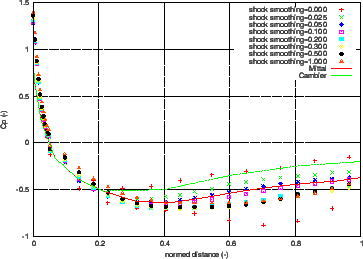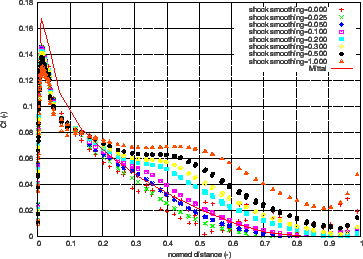A further example is the laminar viscous compressible flow about a naca012 airfoil. Results for this problem were reported by . The entrance Mach number is 0.85, the Reynolds number is 2000. Of interest is the steady state solution. In CalculiX this is obtained by performing a transient CFD-calculation up to steady state. The input deck for this example is called naca012_visc_mach0.85.inp and can be found amoung the CFD test examples. Basing the Reynolds number on the unity chord length of the airfoil, an entrance unity velocity and a entrance unity density leads to a dynamic viscosity of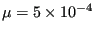. Taking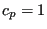and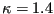leads to a specific gas constant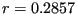(all in consistent units). Use of the entrance Mach number determines the entrance static temperature to be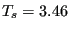. Finally, the ideal gas law leads to a entrance static pressure of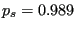. Taking the Prandl number to be 1 determines the heat conductivity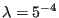. The surface of the airfoil is assumed to be adiabatic.

The results for the pressure and the friction coefficient at the surface of the airfoil are shown in Figures 35 and 36, respectively, as a function of the shock smoothing coefficient. The pressure coefficient is defined by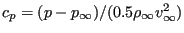, where p is the local static pressure,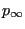,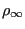and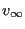are the static pressure, density and velocity at the entrance, respectively. From Figure 35 it is clear that a reduction of the shock smoothing coefficient improves the results. For a zero shock smoothing coefficient, however, the results oscillate and do not make sense any more. Taking into account that the reference results do not totally agree either, a shock smoothing coefficient of 0.025, which is the smallest smoothing coefficient yielding non-oscillating values, leads to the best results. The friction coefficient is defined by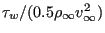, where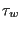is the local shear stress. Here too, a too large shock smoothing coefficient clearly leads to wrong results. A value of 0.05 best agrees with the results by Mittal, however, in the light of the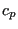-results from the literature a value of 0.025 might be good as well. The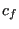-peak at the front of the airfoil is not very well hit: the literature result is 0.17, the CalculiX peak reaches only up to 0.15. While decreasing the shock smoothing coefficient increases the peak, a too coarse mesh density at that location may also play a role. The general advice is to use as little shock smoothing as possible.